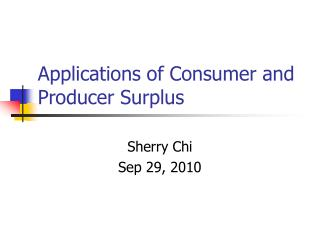DownloadDownload PresentationApplications of Consumer and Producer Surplus

# Applications of Consumer and Producer Surplus

Download Presentation## Applications of Consumer and Producer Surplus

- - - - - - - - - - - - - - - - - - - - - - - - - - - E N D - - - - - - - - - - - - - - - - - - - - - - - - - - -
##### Presentation Transcript

1. Applications of Consumer and Producer Surplus Sherry Chi Sep 29, 2010

2. Consumer Surplus • Consumer Surplus measures the difference between the highest price a consumer is willing to pay and the price the consumer actually pays. • The total amount of consumer surplus in a market is equal to the area below the demand curve and above the market price. See the graph below:

3. P CS Market price=P* Demand Q Q* Consumer Surplus

4. Producer Surplus • Producer surplus is the difference between the lowest price a firm would be willing to accept and the price it actually receives. • The total amount of producer surplus in a market is equal to the area above the market supply curve and below the market price. See the graph below:

5. P Supply Market price=P* PS Q Q* Producer Surplus

6. Efficiency of Competitive Market • Economic Surplus=Consumer Surplus + Producer Surplus • In a perfectly competitive market without government intervention, the total economic surplus is at its maximum. See the graph on the next page:

7. P Supply CS P* PS Demand Q Q* Efficiency of Competitive Market

8. Government Intervention • 1) Price Floor: • Price floor is a legally determined minimum price that sellers may receive. • Usually, price floor is set above the equilibrium price to protect the benefit of sellers.

9. Price Floor • By doing this, the quantity demanded is smaller than the quantity supplied and it will cause surplus in the market. • Ex: In agricultural markets the government imposes a market price for an agricultural product which is higher than the equilibrium price to aid the farmers.

10. P Surplus in the market Supply A Price Floor B C P* E D Demand Q Q* Qs Qd Price Floor • Welfare analysis of price floor:

11. Government Intervention • 2) Price Ceiling: • Price ceiling is legally determined maximum price that sellers may charge. • Price ceiling is usually set below the equilibrium price to protect the benefit of buyers.

12. Price Ceiling • After the price ceiling is imposed, it will cause shortage in the market since the quantity demanded exceed the quantity supplied. Ex: the government rent control policy in the housing market. Such rent control policy put a ceiling on the maximum rent that landlords can charge for an apartment.

13. P Supply A B P* D C Price Ceiling E Demand Shortage Q Q* Qd Qs Price Ceiling

14. Exercises • Problem 1(3.5 Page126) • The graph shows the market for apples. Assume the government has imposed a price floor of \$10 per crate.

15. Exercises

16. Exercises • a. How many crates of apples will be sold after the price floor has been imposed? • Answer: After the price floor has been imposed, 28 million crates of apples are demanded and 34 million crates of apples are supplied. Therefore only 28 million crates of apples will be sold.

17. Exercises • b. Will there be a shortage or a surplus? If there is a shortage or a surplus, how large will it be? • Answer: There will be a surplus in the market since quantity demanded is smaller than quantity supplied. The surplus is 6 millions crates=34- 28.

18. Exercises • c. Will apple producers benefit from the price floor? If so, explain how they will benefit. • Answer: The apple growers will benefit. Their revenue will increase from \$8*30=\$240 million to \$10*28=\$280 million.

19. Exercises • Problem 2: • (4A.7 Page 135) Suppose that you are the vice president of operations of a manufacturing firm that sells an industrial lubricant in a competitive market. Further suppose that your economist gives you the following supply and demand functions:

20. Exercises • Demand: • Supply: • What is the consumer surplus and producer surplus in the market?

21. Exercises

22. Exercises • First, we need to calculate the equilibrium price and quantity. P*=20 and Q*=5. • Then we need to get the intercepts of the two curves. After we write the demand and supply function as that P is a function of Q, demand function becomes P=22.5-0.5Q and supply function becomes P=15+Q. • Then we can see that the two intercepts on the graph is 22.5 and 15.

23. Exercises • CS= (22.5-20)*5*1/2=6.25 • PS= (20-15)*5*1/2= 12.5

24. Exercises • Problem 3:(4A.8 Page 135) • The following graph shows a market in which a price floor of \$3.00 per unit has been imposed. Calculate the values of each of the following.

25. Exercises • A) The deadweight loss • B) The transfer of producer surplus to consumer or the transfer of consumer surplus to producer • C) Producer surplus after the price floor is imposed • D) Consumer surplus after the price floor is imposed

26. Exercises

27. Exercises • Answer: • A) The deadweight loss is the area of C+E=10,000*2*1/2=10,000 • B) B is transfer from consumer surplus to producer surplus. It equals to 10,000*1=10,000

28. Exercises • C) Producer surplus after the price floor is area of B+D+F. It equals to (2+3)*10,000*1/2=25,000 • D) Consumer surplus after the price floor is area of A=10,000*1*1/2=5,000